# Proving Fourier Transform

• I
• henry wang

#### henry wang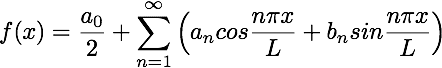Quote: "The Fourier transform is a generalization of the complexFourier series in the limit as [PLAIN]http://mathworld.wolfram.com/images/equations/FourierTransform/Inline1.gif. [Broken] Replace the discrete http://mathworld.wolfram.com/images/equations/FourierTransform/Inline2.gif with the continuous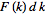while letting [PLAIN]http://mathworld.wolfram.com/images/equations/FourierTransform/Inline4.gif. [Broken] Then change the sum to an integral, and the equations become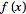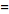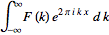(1)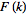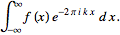"
From: http://mathworld.wolfram.com/FourierTransform.html
Why can we replace A(n) with F(k)dk as L tends to infinity? I know that A(n) will be continues as L tends to infinity, but I can't make sense of F(K)dk.
Can I just let A(n)=F(k)dk or can I proof this from the definition of Fourier series rigorously?

Last edited by a moderator:
Thx I solved it.